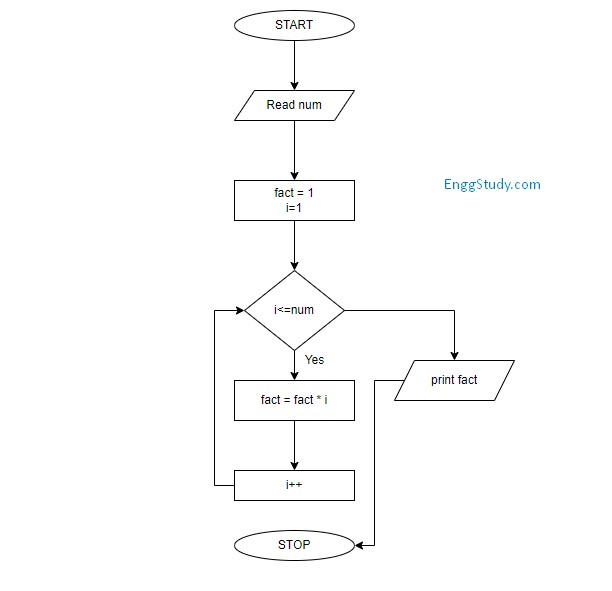# Factorial of a given number – Algorithm, Flowchart, and Program

## What is the factorial of a number?

Factorial is a product of all positive integers less than or equal to a given number n. Factorial is denoted by a symbol (!).  In simple language, factorial is multiplying the whole numbers until 1.

for example,

Factorial of 5! = 5x4x3x2x1 = 120

In this way, we can calculate the factorial of any positive integer number.

In programming, you have to write an algorithm and program to calculate the factorial of a given number. It is one of the primary programs asked during the interview to check your logic-building skills.

Below I have given a flowchart, algorithm, and program to calculate the factorial of a given number.

## Flowchart for finding factorial of a given number## Algorithm for finding factorial of a given number

Step 1: Start

Step 2: Read the input number from the user

Step 2: Declare and initialize variables fact = 1 and i =  1

Step 4:  Repeat the loop until  i<=num

–  fact = fact * i

–  i =  i++

Step 5:  Print fact to get the factorial of a given number

Step 6: Stop

Also Read: Bubble sort algorithm in java

## Factorial of a given number in Java

``````import java.util.Scanner;

class Factorial {

public static void main(String[] args){

Scanner sc = new Scanner(System.in);
System.out.println("Enter Number: ");
int num = sc.nextInt();

int fact = 1;

for(int i=1; i<=num; i++){

fact = fact * i;

}

System.out.println("Factorial of a given number is " + fact);

}

}``````

### 2 thoughts on “Factorial of a given number – Algorithm, Flowchart, and Program”

1.Super

•